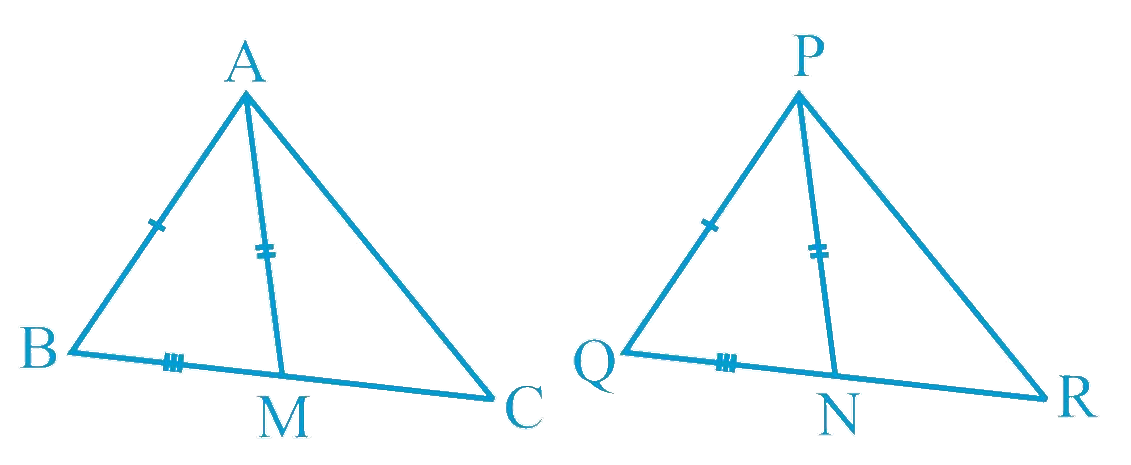# Ex.7.3 Q3 Triangles Solution - NCERT Maths Class 9

Go back to  'Ex.7.3'

## Question

Two sides $$AB$$ and $$BC$$ and median $$AM$$ of one triangle $$ABC$$ are respectively equal to sides $$PQ$$ and $$QR$$ and median $$PN$$ of $$\Delta PQR$$ (see the given figure). Show that:

(i) $$\Delta ABP \cong \Delta PQN$$

(ii) $$\Delta ABC \cong \Delta PQR$$

Video Solution
Triangles
Ex 7.3 | Question 3

## Text Solution

What is Known?

Sides $$AB=PQ$$, $$BC=QR$$ and $$AM = PN$$ also $$AM$$ and $$PN$$ are medians.

To prove:

i) $$\Delta ABM\cong \Delta PQN$$

ii) $$\Delta ABC\cong \Delta PQR$$

Reasoning:

Using the median property we can show Triangles ABM and PQN are congruent by SSS congruency then we can say corresponding parts of congruent triangles are equal and then Triangles ABC and PQR by SAS congruency.Steps:

i) In $$\Delta ABC, AM$$ is the median to $$BC$$.

\begin{align} \therefore BM = \frac{1}{2} BC \end{align}

In $$\Delta PQR, PN$$ is the median to $$QR$$.

\begin{align} \therefore QN = \frac{1}{2} QR \end{align}

However, $$BC = QR$$ [Given]

\begin{align} & \therefore \frac{1}{2}BC = \frac{1}{2}QR\\ & \therefore BM = QN \ldots (1)\end{align}

In $$\Delta ABM$$ and $$\Delta PQN$$,

\begin{align}AB &= PQ\;(\text{Given})\\ BM &= QN\;\\&\text{[From Equation (1)}]\\ AM &= PN\;(\text{Given})\\ \Delta ABM &\cong \Delta PQN \\&\text{(By SSS congruence rule)} \end{align}

\begin{align}& \angle ABM = \angle PQN \;(\text{By }CPCT)\\ &\angle ABC = \angle PQR\dots (2) \end{align}

ii) In $$\Delta ABC$$ and $$\Delta PQR$$,

\begin{align}AB &= PQ\;(\text{Given})\\ \angle ABC &= \angle PQR \\&\text{[From Equation (2)}]\\\\BC &= QR\;(\text{Given})\\ \Delta ABC &\cong \Delta PQR \\&(\text{By SAS congruence rule})\end{align}

Video Solution
Triangles
Ex 7.3 | Question 3

Learn from the best math teachers and top your exams

• Live one on one classroom and doubt clearing
• Practice worksheets in and after class for conceptual clarity
• Personalized curriculum to keep up with school